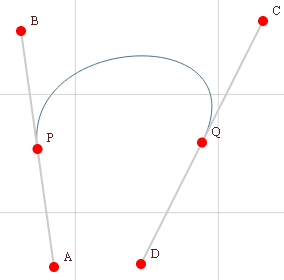Search IntMath
Close

# Interactive Bezier Curve Graphs

By Murray Bourne, 02 Jan 2013

I recently added a new interactive graph on the Mathematics of Vector Art page.

Vector art is made up of a collection of primitives. These primitives include simple geometric figures like lines, triangles, squares, circles and so on.

We can also have quadratic curves (parabolas) and cubic curves.

Bezier (or more correctly, Bézier) Curves are also used in vector art. These curves help us to join points with smooth curves.Screen shot from the interactive.

The interactive lets you explore straight lines, parabolas, cubic curves and Bezier Curves.

The link again: Mathematics of Vector Art

### Comment Preview

HTML: You can use simple tags like <b>, <a href="...">, etc.

To enter math, you can can either:

1. Use simple calculator-like input in the following format (surround your math in backticks, or qq on tablet or phone):
a^2 = sqrt(b^2 + c^2)
(See more on ASCIIMath syntax); or
2. Use simple LaTeX in the following format. Surround your math with $$ and $$.
$$\int g dx = \sqrt{\frac{a}{b}}$$
(This is standard simple LaTeX.)

NOTE: You can mix both types of math entry in your comment.